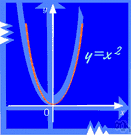# algebra

(redirected from Algebra 1)
Also found in: Thesaurus, Encyclopedia.

## al·ge·bra

(ăl′jə-brə)
n.
1. A branch of mathematics in which symbols, usually letters of the alphabet, represent numbers or members of a specified set and are used to represent quantities and to express general relationships that hold for all members of the set.
2. A set together with a pair of binary operations defined on the set. Usually, the set and the operations simultaneously form both a ring and a module.

[Middle English, bone-setting, and Italian, algebra, both from Medieval Latin, from Arabic al-jabr (wa-l-muqābala), the restoration (and the compensation), addition (and subtraction) : al-, the + jabr, bone-setting, restoration (from jabara, to set (bones), force, restore; see gpr in Semitic roots).]

al′ge·bra′ist (-brā′ĭst) n.

## algebra

(ˈældʒɪbrə)
n
1. (Mathematics) a branch of mathematics in which arithmetical operations and relationships are generalized by using alphabetic symbols to represent unknown numbers or members of specified sets of numbers
2. the branch of mathematics dealing with more abstract formal structures, such as sets, groups, etc
[C14: from Medieval Latin, from Arabic al-jabr the bone-setting, reunification, mathematical reduction]
algebraist n

## al•ge•bra

(ˈæl dʒə brə)

n.
1. the branch of mathematics that deals with general statements of relations, utilizing letters and other symbols to represent specific sets of numbers, values, vectors, etc., in the description of such relations.
2. any system of notation adapted to the study of a special system of relationship: algebra of classes.
[1535–45; < Medieval Latin < Arabic al-jabr literally, restoration]
al`ge•bra′ist (-ˈbreɪ ɪst) n.

## al·ge·bra

(ăl′jə-brə)
A branch of mathematics dealing with the relations and properties of quantities. It uses letters and other symbols to represent numbers, especially in equations to solve problems or to express general mathematical relationships.
Word History For much of the Middle Ages, the center of scientific learning was not Europe, but the Islamic world of the Arabs. The Arabs studied the Greek classics of Plato and Aristotle while Europe nearly forgot about them. The Arabs were particularly interested in medicine and astronomy, and because astronomy requires making careful measurements and calculations, they became expert mathematicians. In the 800s, an Arabic mathematician named Muhammad al-Khwarizmi wrote a book called The Book of Restoring and Balancing, which explained the principles of algebra. Algebra had been developed earlier by mathematicians in Greece and India, but al-Khwarizmi's book, as the first comprehensive treatment of it, became a medieval bestseller. The Arabic word for "the restoring" in the book's title is al-jabr, which is the source of our word algebra. Al-Khwarizmi's own name is the source of another mathematical term in English, algorithm.

## algebra

the branch of mathematics that treats the representation and manip-ulation of relationships among numbers, values, vectors, etc. — algebraic, adj.
ThesaurusAntonymsRelated WordsSynonymsLegend:
 Noun 1algebra - the mathematics of generalized arithmetical operationstransposition - (mathematics) the transfer of a quantity from one side of an equation to the other along with a change of signmath, mathematics, maths - a science (or group of related sciences) dealing with the logic of quantity and shape and arrangementpure mathematics - the branches of mathematics that study and develop the principles of mathematics for their own sake rather than for their immediate usefulnessquadratics - a branch of algebra dealing with quadratic equationslinear algebra - the part of algebra that deals with the theory of linear equations and linear transformationvector algebra - the part of algebra that deals with the theory of vectors and vector spacesmatrix algebra - the part of algebra that deals with the theory of matrices
Translations
جَبْر، عِلْم الجَبْر
algebra
algebrabogstavregning
algebra
algebra
algebra
algebra
algebra
algebraalgebrinis
algebra
algebra
algebra

[ˈældʒɪbrə] N

[ˈældʒɪbrə] n

nAlgebra f

## algebra

[ˈældʒɪbrə] nalgebra

## algebra

(ˈӕldʒibrə) noun
a method of calculating using letters and signs to represent numbers.